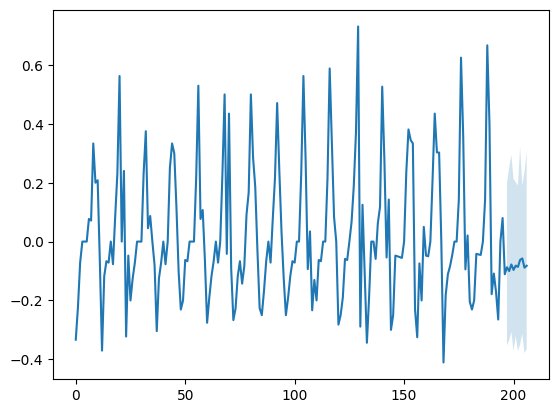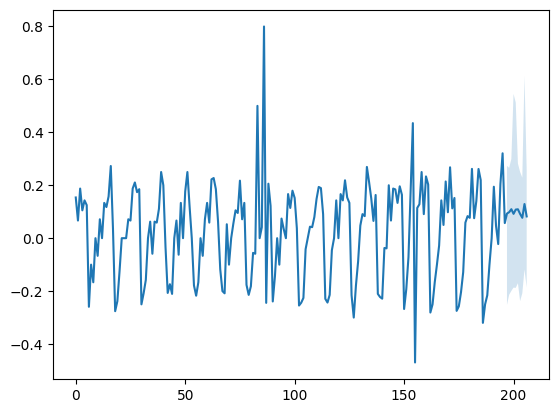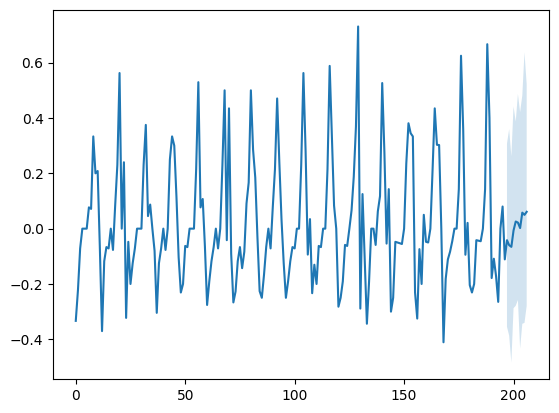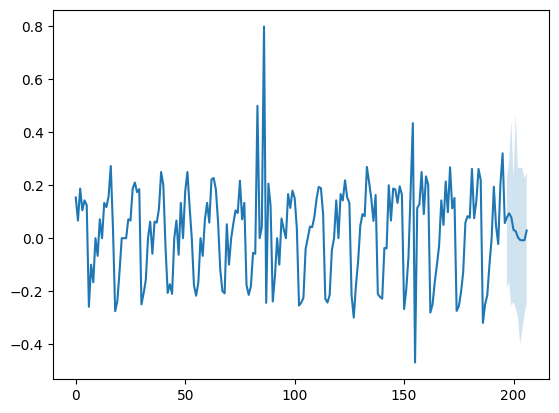A new Python version of ahead, v0.9.0 is now available on GitHub and PyPI.

ahead is a Python and R package for univariate and multivariate time series forecasting, with uncertainty quantification (in particular, simulation-based uncertainty quantification).

Here are the new features in v0.9.0:

• progress bars for possibly long calculations: the bootstrap (independent, circular block, moving block)

• plot for Ridge2Regressor (a work in progress, still needs to use series names, and display dates correctly, for all classes, not just Ridge2Regressor)

Since this implementation is based on the R version, it could take some time to import R packages when using Python’s ahead for the first time. There’s something new regarding this situation (well… ha ha): R packages are now installed on the fly. Meaning: only when they’re required.

# Example 1

Start by installing ahead v0.9.0:

pip install ahead

import numpy as np
import pandas as pd
from time import time
from ahead import Ridge2Regressor # this is where the R packages are installed (if not available in the environment, and ONLY the 1st time)

url = "https://raw.githubusercontent.com/thierrymoudiki/mts-data/master/heater-ice-cream/ice_cream_vs_heater.csv"

df.set_index('Month', inplace=True) # only for ice_cream_vs_heater
df.index.rename('date') # only for ice_cream_vs_heater

df = df.pct_change().dropna()

regr1 = Ridge2Regressor(h = 10, date_formatting = "original",
type_pi="rvinecopula",
margins="empirical",
B=50, seed=1)

regr1.forecast(df) # this is where the R packages are installed (if not available in the environment, and ONLY the 1st time)

regr1.plot(0) # dates are missing, + want to use series names
regr1.plot(1)# Example 2

regr2 = Ridge2Regressor(h = 10, date_formatting = "original",
type_pi="movingblockbootstrap",
B=50, seed=1)

regr2.forecast(df) # a progress bar is displayed

regr2.plot(0) # dates are missing, + want to use series names
regr2.plot(1)